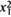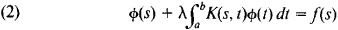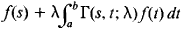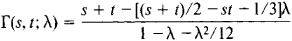# Resolvent

Also found in: Dictionary, Thesaurus, Medical, Wikipedia.
Related to Resolvent: resolution, Resolvent kernel

## resolvent

[ri′zäl·vənt]
(mathematics)
For a linear operator T on a Banach space, the function, defined on the complement of the spectrum of T given by (T- λ I)-1 for each λ in this complement, where I is the identity operator; this enables a study of T relative to its eigenvalues.
McGraw-Hill Dictionary of Scientific & Technical Terms, 6E, Copyright © 2003 by The McGraw-Hill Companies, Inc.
The following article is from The Great Soviet Encyclopedia (1979). It might be outdated or ideologically biased.

## Resolvent

a mathematical term with various meanings. We speak, for example, of resolvent equations, resolvent kernels, and resolvent operators.

In algebra, the term “resolvent” is used in several senses. Thus, by the resolvent of the algebraic equation f(x) = 0 of degree n we mean an algebraic equation g(x) = 0 such that its coefficients are rational functions of the coefficients of f(x) and, if the roots of this equation are known, it is possible to find the roots of f(x) = 0 by solving simpler equations of degree at most n. For example, the equation

ν3 - a2v2 + (a1a3 - 4α4) ν - (α\αΛ - 2 αΛ + a]) = 0

is one of the cubic resolvents of the fourth-degree equation

(1) x4 + a1x3 + a2x2 + a3x + a4 = 0

If ν1, v2, and v3 are the roots of the resolvent equation, the roots x1, x2, x3, and x4 of equation (1) can be found by solving the quadratic equations σ2 - vk σ + a4 = 0, k = 1, 2, 3. Thus, if xn and nk are the roots of these quadratic equations, x1x2= x3x4 = n, x1x3 = x2x2x4 = x2x2x4 = n3,= ξ1x2/n3, and so on. A Galois resolvent of the equation f(x) is an algebraic equation g(x) = 0 irreducible over a given field (seeGALOIS THEORY) such that when one of its roots is adjoined to the field, there results a field containing all the roots of the equation f(x) = 0.

The term “resolvent” is used in a somewhat different sense in what is known as the Hilbert-Chebotarev resolvent problem.

In the theory of integral equations, the resolvent of the equationis a function Γ (s, t; λ) of the variables s and t and the parameter λ such that the solution of equation (2) can be represented in the formprovided that λ is not an eigenvalue of equation (2). For example, the resolvent of the kernel K(s, t) = S + t is the functionIn the theory of linear operators, the resolvent of the operator A is the family of operators Rλ = (A - λ E)-1 where the complex parameter λ takes on any values outside the spectrum of A.